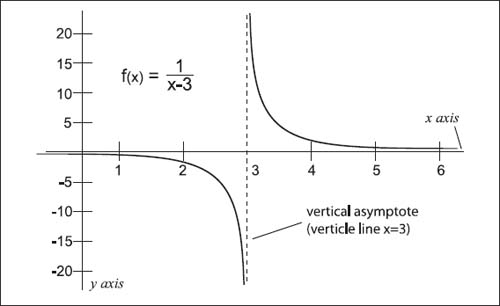NextPrevious

# What are vertical asymptotes in association with limits?

Using the example above, as x gets closer to 3, the points on the graph get closer to the vertical line x = 3 (seen as a dashed line on the graph below). This line is called a vertical asymptote. The following charts shows four of the vertical asymptotes for the given function f(x) and their notations.Graph of an equation with infinity limited by x = 3.

Close

This is a web preview of the "The Handy Math Answer Book" app. Many features only work on your mobile device. If you like what you see, we hope you will consider buying. Get the App